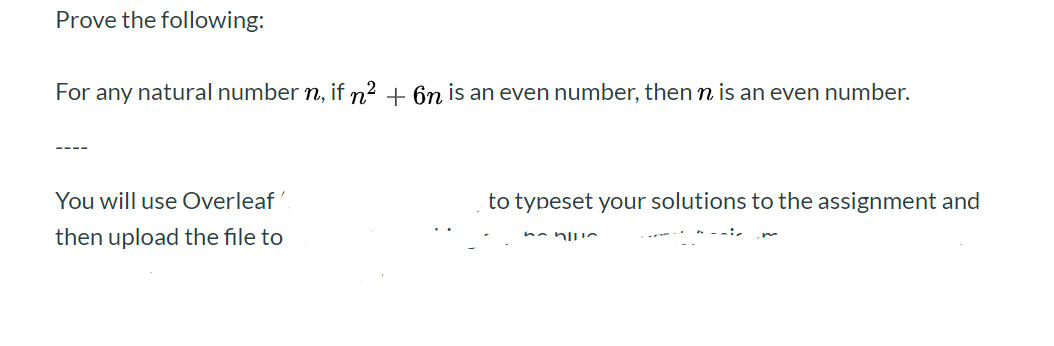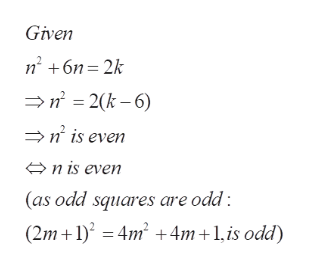Prove the following:For any natural number n, if n2 6n is an even number, then n is an even number.-You will use Overleafto typeset your solutions to the assignment andthen upload the file to

Question

Prove the following:

For any natural number n, if n 2 + 6 n is an even number, then n is an even number.help_outlineImage TranscriptioncloseProve the following: For any natural number n, if n2 6n is an even number, then n is an even number. - You will use Overleaf to typeset your solutions to the assignment and then upload the file to fullscreen
Step 1

To prve that n is an even integer under the given conditions

Step 2

Proof uses the property that the squ...help_outlineImage TranscriptioncloseGiven n62k n 2(k-6) > is even n is even (as odd squares are odd (2m 1)4m2 4m 1,is odd) fullscreen

Want to see the full answer?

See Solution

Want to see this answer and more?

Our solutions are written by experts, many with advanced degrees, and available 24/7

See Solution
Tagged in

Math